Sequences and Series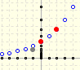Geometric sequences graphic representations. Sum of terms of a geometric sequence and geometric series.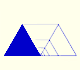One intuitive example of how to sum a geometric series. A geometric series of ratio less than 1 is convergent.The geometric series of ratio 1/2 is convergent. We can represent this series using a rectangle and cut it in half successively. Here we use a rectangle such us all rectangles are similar.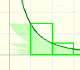Using a decreasing positive function you can define series. The integral test is a tool to decide if a series converges o diverges. If a series converges, the integral test provide us lower and upper bounds.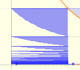Gamma, the Euler's constant, is defined using a covergent series. Powers and PolynomialsTwo points determine a stright line. As a function we call it a linear function. We can see the slope of a line and how we can get the equation of a line through two points. We study also the x-intercept and the y-intercept of a linear equation.Power with natural exponents are simple and important functions. Their inverse functions are power with rational exponents (a radical or a nth root)Polynomials of degree 2 are quadratic functions. Their graphs are parabolas. To find the x-intercepts we have to solve a quadratic equation. The vertex of a parabola is a maximum of minimum of the function.Polynomials of degree 3 are cubic functions. A real cubic function always crosses the x-axis at least once.We can consider the polynomial function that passes through a series of points of the plane. This is an interpolation problem that is solved here using the Lagrange interpolating polynomial. Rational FunctionsRational functions can be writen as the quotient of two polynomials. Linear rational functions are the simplest of this kind of functions.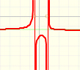When the denominator of a rational function has degree 2 the function can have two, one or none real singularities.For large absolute values of x, some rational functions behave like an oblique straight line, we call this line an oblique or slant asymptote.You can add a polynomial to a proper rational function. The end behavior of this rational function is very similar to the polynomial. Polynomial functions and derivativeThe derivative of a lineal function is a constant function.The derivative of a quadratic function is a linear function, it is to say, a straight line.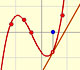The derivative of a cubic function is a quadratic function, a parabola.Lagrange polynomials are polynomials that pases through n given points. We use Lagrange polynomials to explore a general polynomial function and its derivative.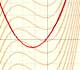If the derivative of F(x) is f(x), then we say that an indefinite integral of f(x) with respect to x is F(x). We also say that F is an antiderivative or a primitive function of f. IntegralThe integral concept is associate to the concept of area. We began considering the area limited by the graph of a function and the x-axis between two vertical lines.If we consider the lower limit of integration a as fixed and if we can calculate the integral for different values of the upper limit of integration b then we can define a new function: an indefinite integral of f.Monotonic functions in a closed interval are integrable. In these cases we can bound the error we make when approximating the integral using rectangles.The integral of power functions was know by Cavalieri from n=1 to n=9. Fermat was able to solve this problem using geometric progressions.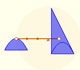Archimedes show us in 'The Method' how to use the lever law to discover the area of a parabolic segment.Studying the volume of a barrel, Kepler solved a problem about maxima in 1615. Polynomial functions and integral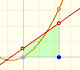It is easy to calculate the area under a straight line. This is the first example of integration that allows us to understand the idea and to introduce several basic concepts: integral as area, limits of integration, positive and negative areas.To calculate the area under a parabola is more difficult than to calculate the area under a linear function. We show how to approximate this area using rectangles and that the integral function of a polynomial of degree 2 is a polynomial of degree 3.We can see some basic concepts about integration applied to a general polynomial function. Integral functions of polynomial functions are polynomial functions with one degree more than the original function. The Fundamental Theorem of CalculusThe Fundamental Theorem of Calculus tell us that every continuous function has an antiderivative and shows how to construct one using the integral.The Second Fundamental Theorem of Calculus is a powerful tool for evaluating definite integral (if we know an antiderivative of the function). Piecewise FunctionsAs an introduction to Piecewise Linear Functions we study linear functions restricted to an open interval: their graphs are like segments.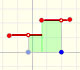A piecewise function is a function that is defined by several subfunctions. If each piece is a constant function then the piecewise function is called Piecewise constant function or Step function.A continuous piecewise linear function is defined by several segments or rays connected, without jumps between them.Graphs of these functions are made of disconnected line segments. There are points where a small change in x produces a sudden jump in the value of the function. Exponentials and LogarithmsWe can study several properties of exponential functions, their derivatives and an introduction to the number e.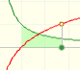Using the integral of the equilateral hyperbola we can define a new function that is the natural logarithm function.The natural logaritm can be defined using the integral of the rectangular hiperbola. In this page we are going to see an important property of this integral. Using this property you can justify that the logarithm of a product is the sum of the logarithms.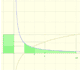The main property of a logarithm function is that the logarithm of a product is the sum of the logarithms of the individual factors.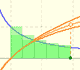The logarithm of the number e is equal to 1. Using this definition of the number e we can approximate its value.Constant e is the number whose natural logarithm is 1. It can be defined as a limit of a sequence related with the compound interest. Both definitions for e are equivalent.After the definition of the natural logarithm function as an integral you can define the exponential function as the inverse function of the logarithm.Different hyperbolas allow us to define different logarithms functions and their inversas, exponentials functions.Mercator published his famous series for the Logarithm Function in 1668. Euler discovered a practical series to calculate.Taylor's PolynomialsBy increasing the degree, Taylor polynomial approximates the exponential function more and more.Mercator published his famous series for the Logarithm Function in 1668. Euler discovered a practical series to calculate.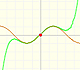By increasing the degree, Taylor polynomial approximates the sine function more and more.The function is not defined for values less than -1. Taylor polynomials about the origin approximates the function between -1 and 1.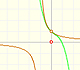The function has a singularity at -1. Taylor polynomials about the origin approximates the function between -1 and 1.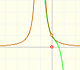The function has a singularity at -1. Taylor polynomials about the origin approximates the function between -1 and 1.This function has two real singularities at -1 and 1. Taylor polynomials approximate the function in an interval centered at the center of the series. Its radius is the distance to the nearest singularity.This is a continuos function and has no real singularities. However, the Taylor series approximates the function only in an interval. To understand this behavior we should consider a complex function.